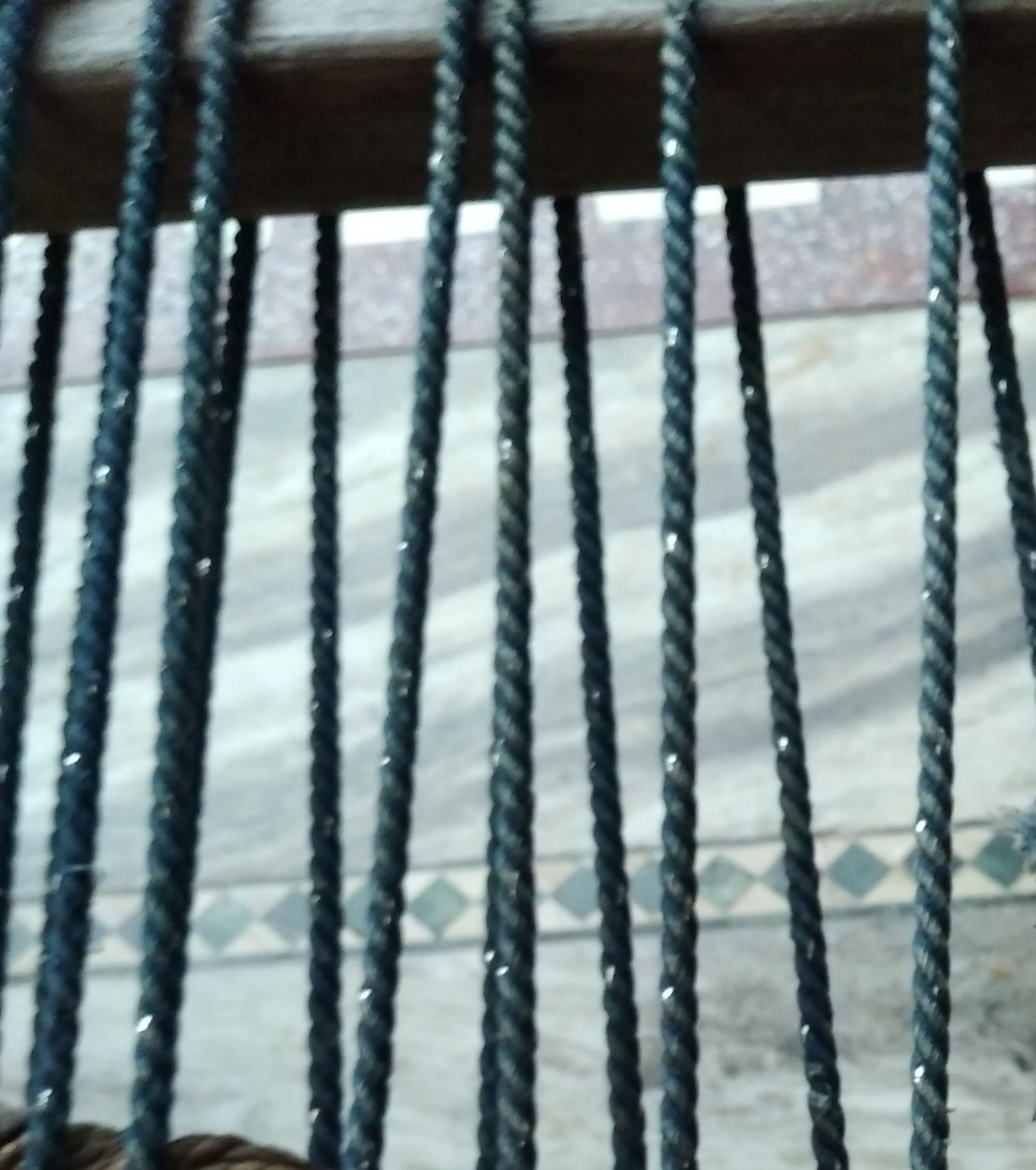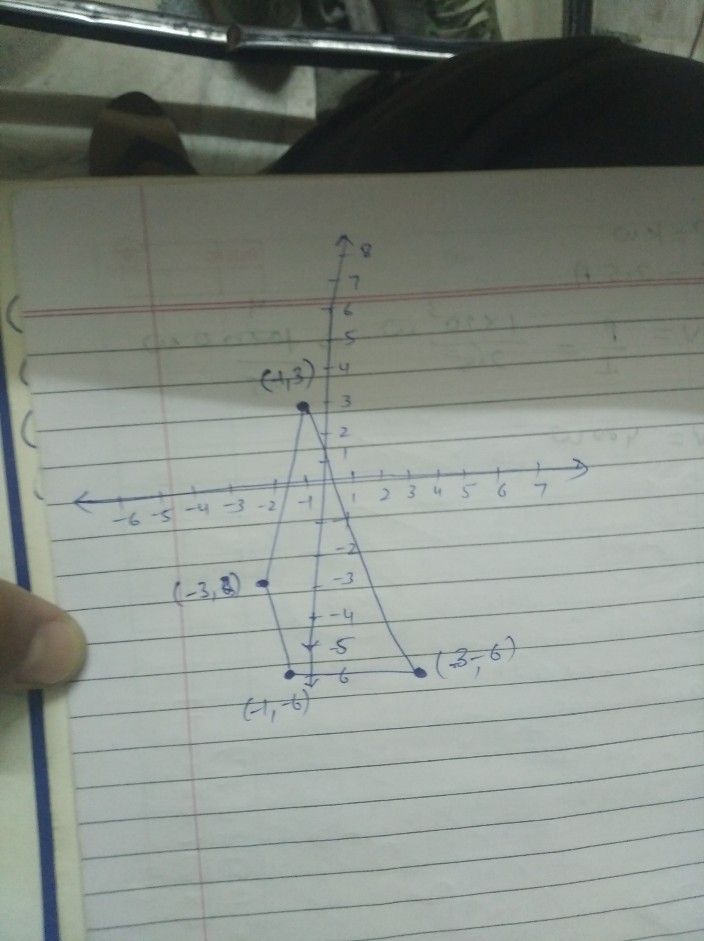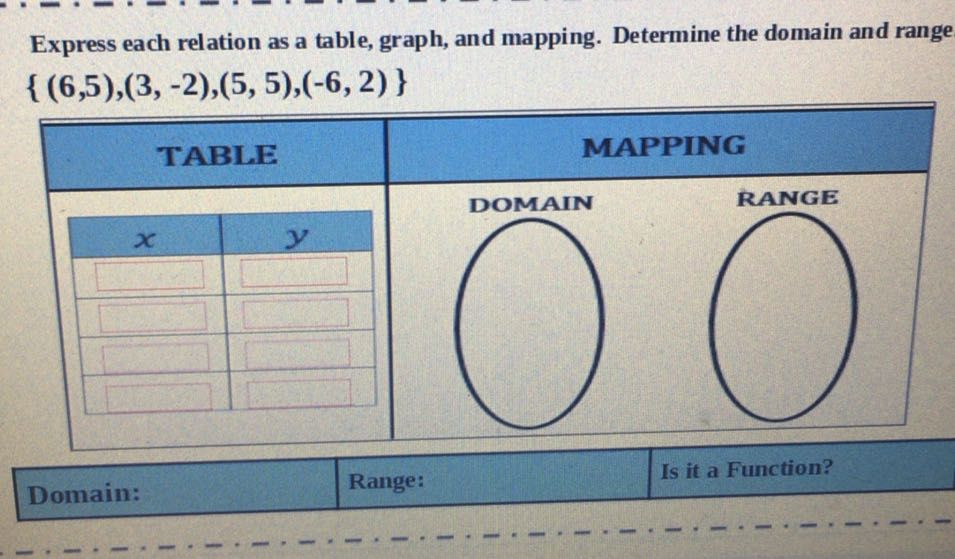Symbol
Problem$y$ A $6$ Graph the Points: $5$ $4$ $3$ $2$ $\left(\left(-1,3\right),\left(3,-6\right),\left(-1,-6\right),\left(-3,-3\right)\right)$ $°$ $2-1-1$ $1$ $234$ $5$ $56$ $\bar{-6-5}$ $4-3.2-1$ $-29$ $3$ $4$ $3$ $5$ $61$
Algebra
SolutionQanda teacher - shivasiryou understand this solutionStudentI need help
With thisQanda teacher - shivasirStudent
OkeyQanda teacher - shivasir
sorry this is anothet question soStudent
YeaQanda teacher - shivasir
so this is qunda rule against
sorry